# Coordination compounds Questions and Answers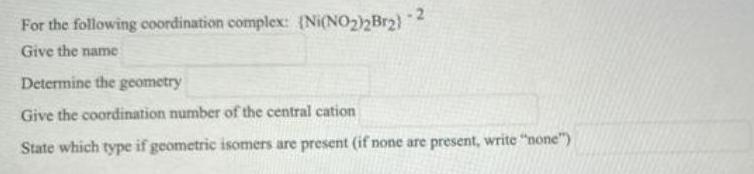Inorganic Chemistry
Coordination compounds
For the following coordination complex: (Ni(NO₂)₂Br₂)^-2 Give the name Determine the geometry Give the coordination number of the central cation State which type if geometric isomers are present (if none are present, write "none")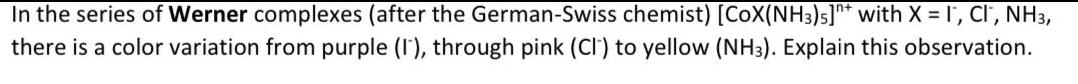Inorganic Chemistry
Coordination compounds
In the series of Werner complexes (after the German-Swiss chemist) [CoX(NH3)5]n* with X = I™, CI', NH3, there is a color variation from purple (1), through pink (CI) to yellow (NH3). Explain this observation.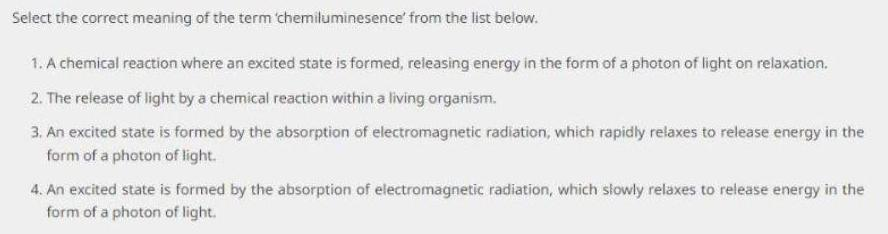Inorganic Chemistry
Coordination compounds
Select the correct meaning of the term 'chemiluminesence' from the list below. 1. A chemical reaction where an excited state is formed, releasing energy in the form of a photon of light on relaxation. 2. The release of light by a chemical reaction within a living organism. 3. An excited state is formed by the absorption of electromagnetic radiation, which rapidly relaxes to release energy in the form of a photon of light. 4. An excited state is formed by the absorption of electromagnetic radiation, which slowly relaxes to release energy in the form of a photon of light.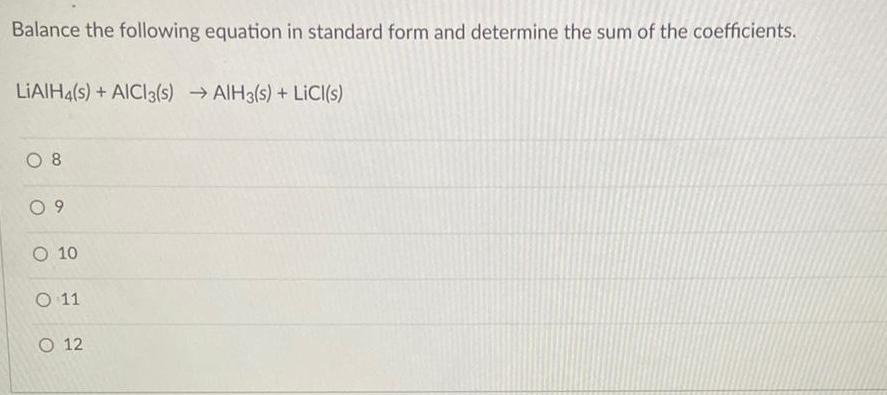Inorganic Chemistry
Coordination compounds
Balance the following equation in standard form and determine the sum of the coefficients. LIAIH4(s) + AICI3(s) →AIH3(s) + LiCl(s) 8 9 10 11 12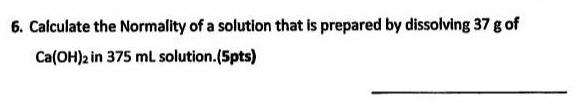Inorganic Chemistry
Coordination compounds
Calculate the Normality of a solution that is prepared by dissolving 37 g of Ca(OH)2 in 375 ml solution.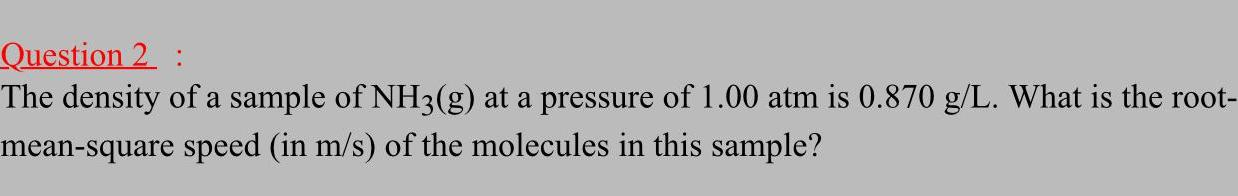Inorganic Chemistry
Coordination compounds
The density of a sample of NH3(g) at a pressure of 1.00 atm is 0.870 g/L. What is the root- mean-square speed (in m/s) of the molecules in this sample?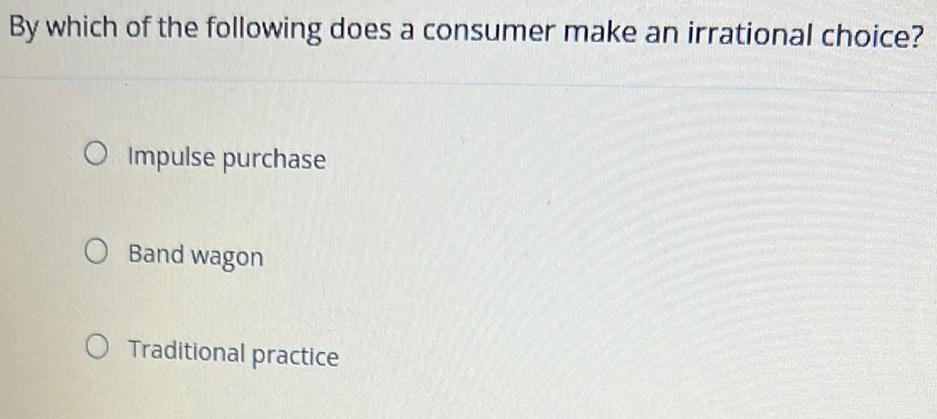Inorganic Chemistry
Coordination compounds
By which of the following does a consumer make an irrational choice? Impulse purchase Band wagon Traditional practice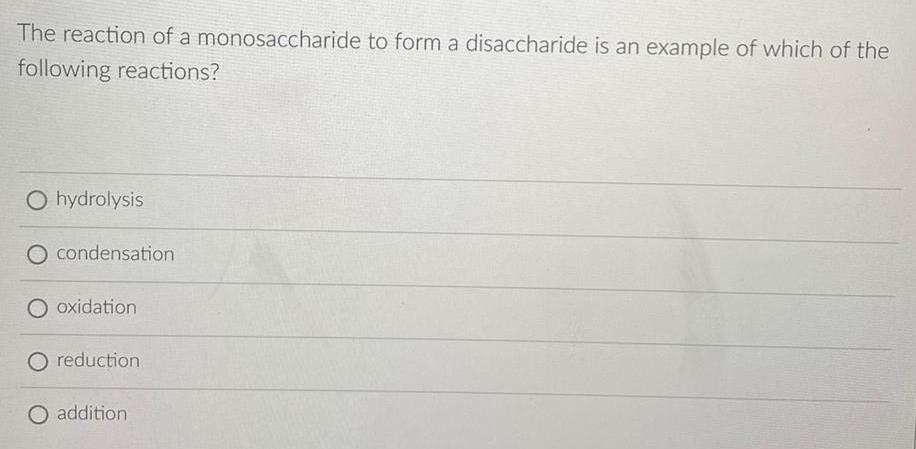Inorganic Chemistry
Coordination compounds
The reaction of a monosaccharide to form a disaccharide is an example of which of the following reactions? hydrolysis condensation oxidation reduction addition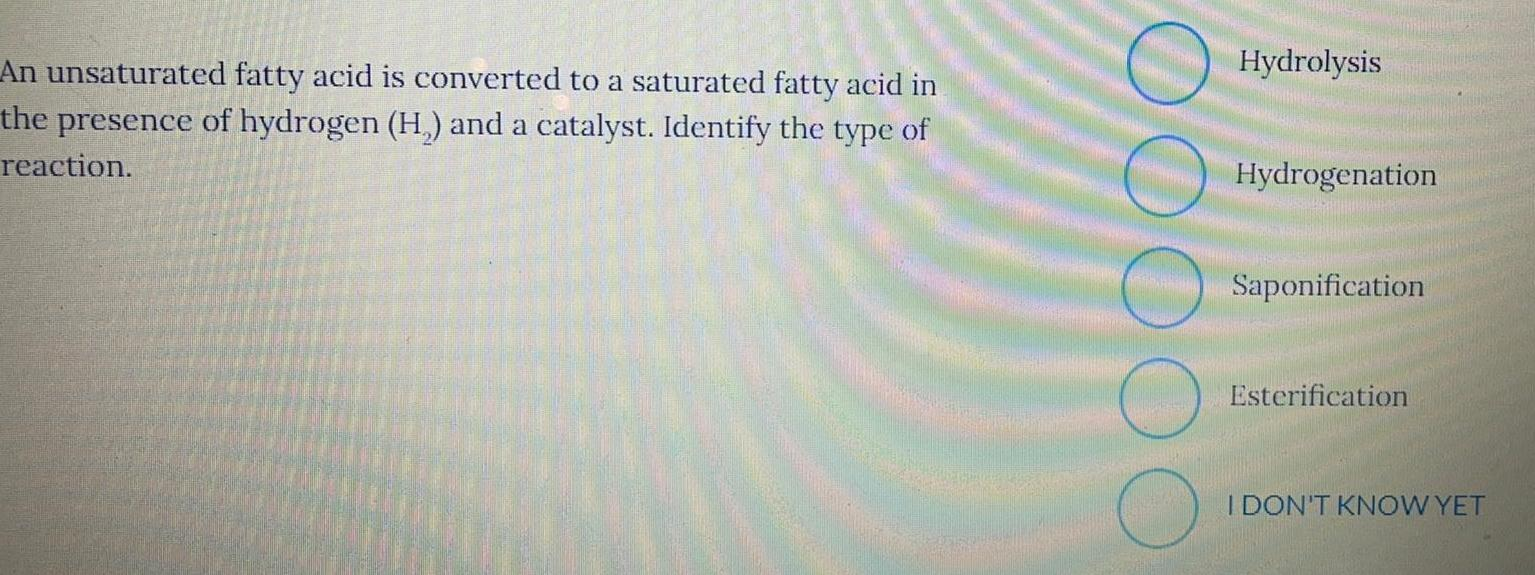Inorganic Chemistry
Coordination compounds
An unsaturated fatty acid is converted to a saturated fatty acid in the presence of hydrogen (H₂) and a catalyst. Identify the type of reaction. Hydrolysis Hydrogenation Saponification Esterification I DON'T KNOW YET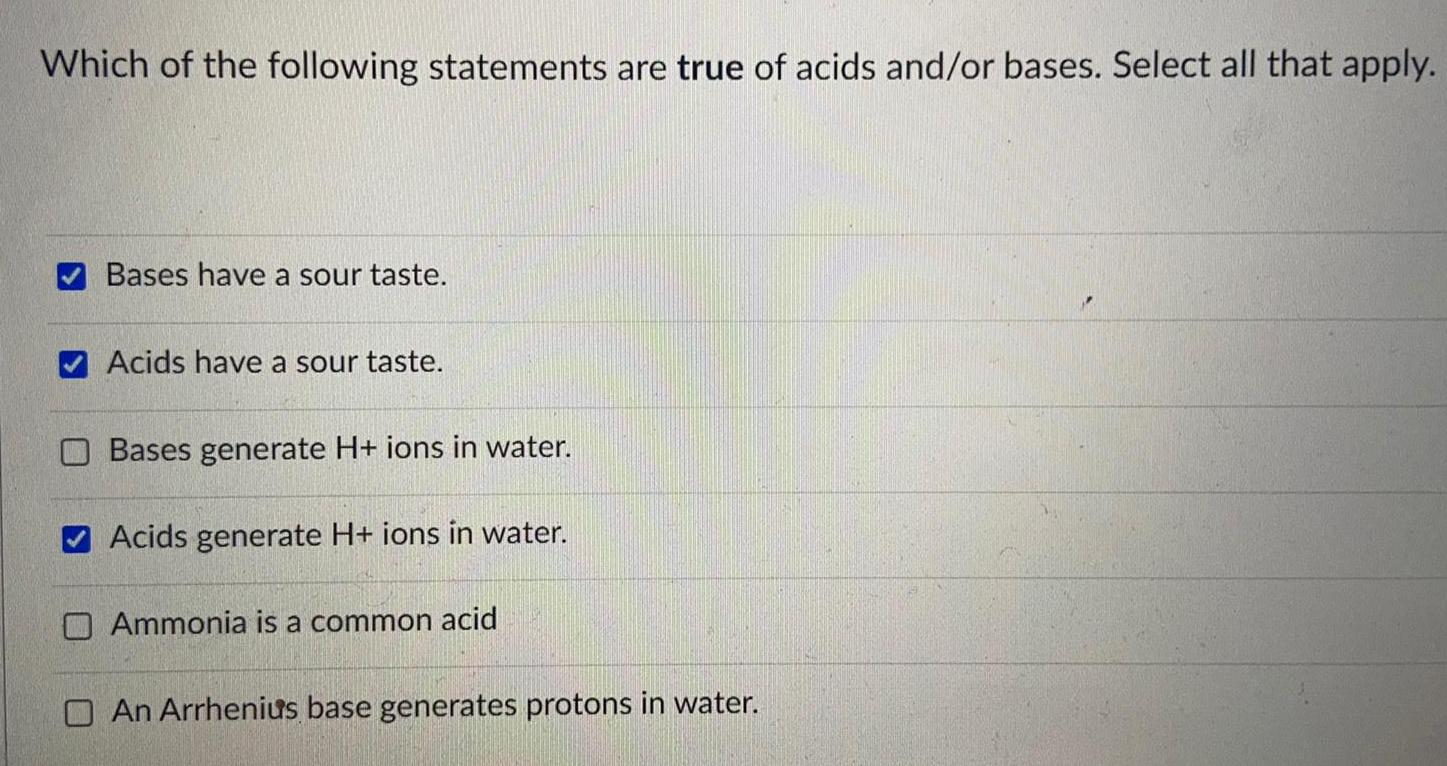Inorganic Chemistry
Coordination compounds
Which of the following statements are true of acids and/or bases. Select all that apply. Bases have a sour taste. Acids have a sour taste. Bases generate H+ ions in water. Acids generate H+ ions in water. Ammonia is a common acid An Arrhenius base generates protons in water.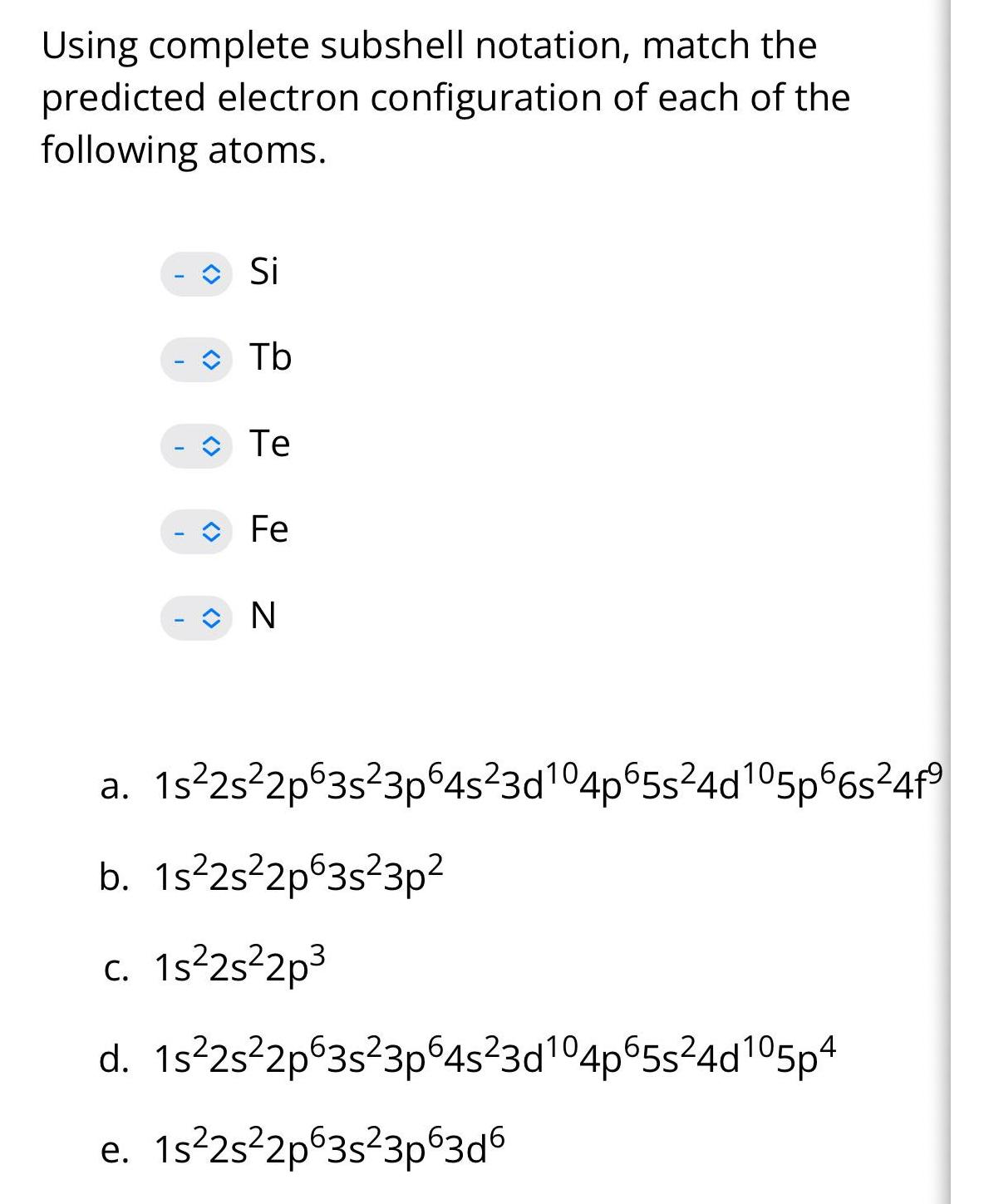Inorganic Chemistry
Coordination compounds
Using complete subshell notation, match the each the predicted electron configuration of following atoms. Si Tb Te Fe N a. 1s²2s²2p63s²3p64s²3d¹04p65s²4d105p66s²4f⁹ b. 1s²2s²2p63s²3p² c. 1s²2s²2p³ d. 1s²2s²2p63s²3p64s²3d¹04p65s²4d105p4 e. 1s²2s²2p63s²3p63d6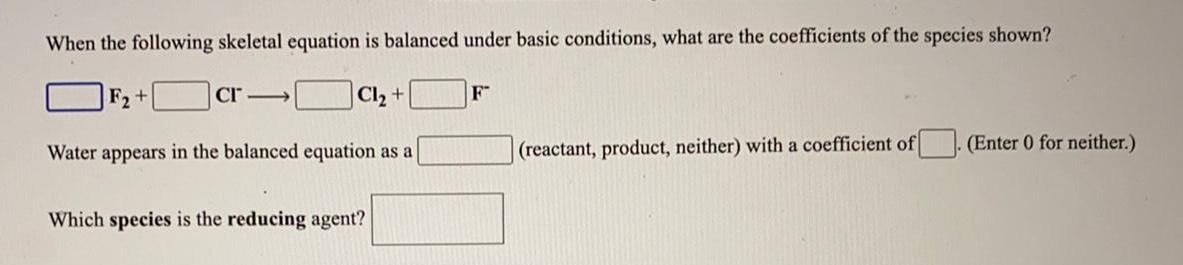Inorganic Chemistry
Coordination compounds
When the following skeletal equation is balanced under basic conditions, what are the coefficients of the species shown? F2 + CI Cl₂ + F- Water appears in the balanced equation as a(reactant, product, neither) with a coefficient of(Enter 0 for neither.) Which species is the reducing agent?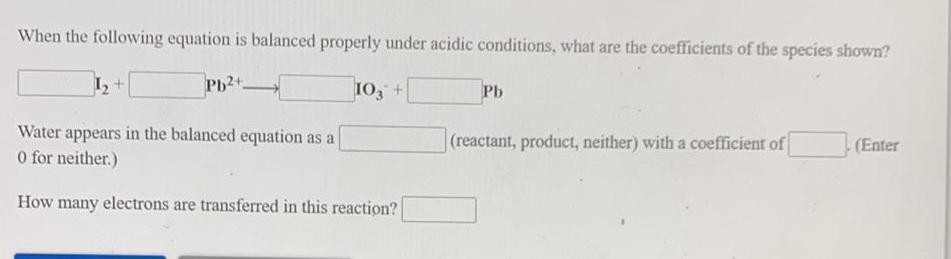Inorganic Chemistry
Coordination compounds
When the following equation is balanced properly under acidic conditions, what are the coefficients of the species shown? I2 + Pb2+ ---> IO3- + Pb Water appears in the balanced equation as a (reactant, product, neither) with a coefficient of 0 for neither.) How many electrons are transferred in this reaction?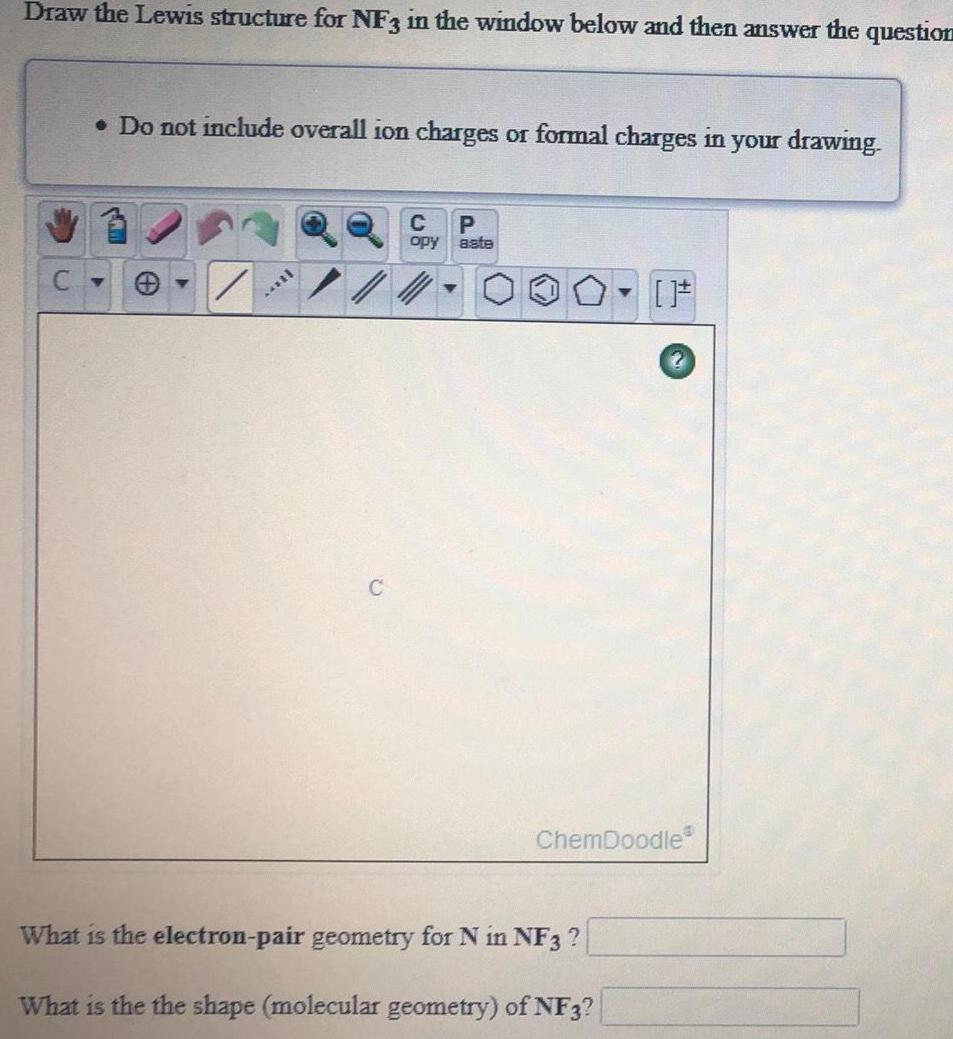Inorganic Chemistry
Coordination compounds
Draw the Lewis structure for NF3 in the window below and then answer the question Do not include overall ion charges or formal charges in your drawing. What is the electron-pair geometry for N in NF3 ? What is the the shape (molecular geometry) of NF3?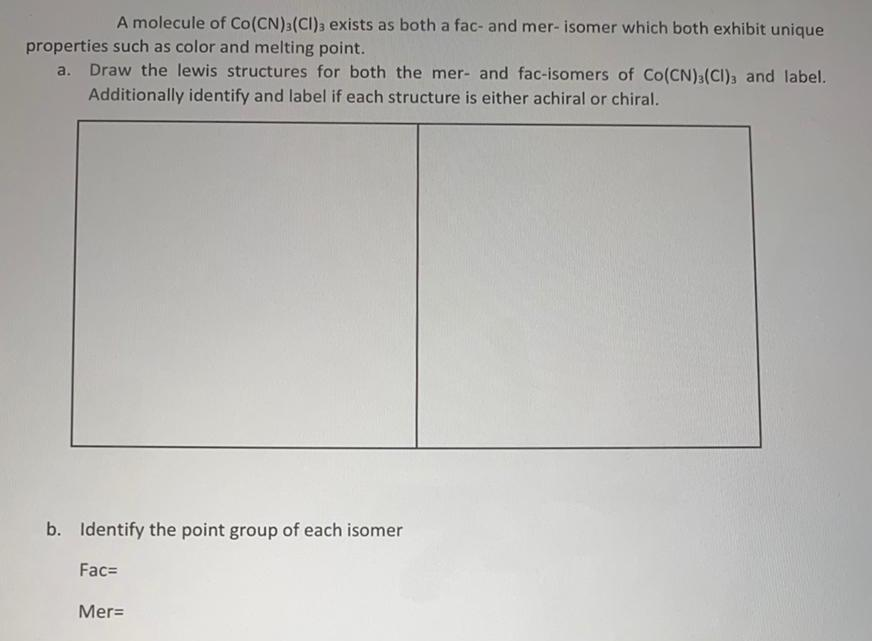Inorganic Chemistry
Coordination compounds
A molecule of Co (CN) 3 (CI)3 exists as both a fac- and mer- isomer which both exhibit unique properties such as color and melting point. a. Draw the lewis structures for both the mer- and fac-isomers of Co(CN)3(CI)3 and label. Additionally identify and label if each structure is either achiral or chiral. b. Identify the point group of each isomer Fac= Mer=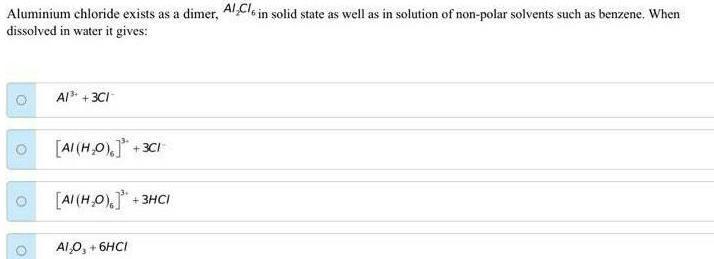Inorganic Chemistry
Coordination compounds
Aluminium chloride exists as a dimer, Al2Cl6 in solid state as well as in solution of non-polar solvents such as benzene. When dissolved in water it gives: